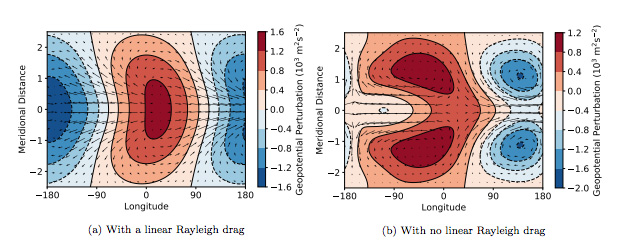# The Equatorial Jet Speed on Tidally Locked Planets: Terrestrial Planets

Press Release
astro-ph.EP
September 1, 2020
Filed underThe geopotential and velocity fields at the p = 0.39 bar (z ≈ 8630 m) level for the stationary solutions to Equation 5. This level corresponds to the peak of the “Stationary Horizontal” acceleration in Figure 3. The solution in the first plot has a linear drag αdynu for a drag timescale τdyn = 1/αdyn = 1d, and the second plot has no linear drag. Away from the equator, where the momentum balance is governed by the Coriolis force, the two solutions are similar. Near the equator, the solutions are governed by linear drag and nonlinear balance respectively. This distinction is vital to accurately calculating the equatorial velocities and acceleration.

The atmospheric circulation of tidally locked planets is dominated by a superrotating eastward equatorial jet.

We develop a predictive theory for the formation of this jet, proposing a mechanism in which the three-dimensional stationary waves induced by the day-night forcing gradient produce an equatorial acceleration. This is balanced in equilibrium by an interaction between the resulting jet and the vertical motion of the atmosphere. The three-dimensional structure of the zonal acceleration is vital to this mechanism.

We demonstrate this mechanism in a hierarchy of models. We calculate the three-dimensional stationary waves induced by the forcing on these planets, and show the vertical structure of the zonal acceleration produced by these waves, which we use to suggest a mechanism for how the jet forms. GCM simulations are used to confirm the equilibrium state predicted by this mechanism, where the acceleration from these waves is balanced by an interaction between the zonal-mean vertical velocity and the jet. We derive a simple model of this using the “Weak Temperature Gradient” approximation, which gives an estimate of the jet speed on a terrestrial tidally locked planet.

We conclude that the proposed mechanism is a good description of the formation of an equatorial jet on a terrestrial tidally locked planet, and should be useful for interpreting observations and simulations of these planets. The mechanism requires assumptions such as a large equatorial Rossby radius and weak acceleration due to transient waves, and a different mechanism may produce the equatorial jets on gaseous tidally locked planets.

Mark Hammond, Shang-Min Tsai, Raymond T. Pierrehumbert